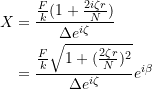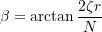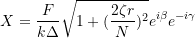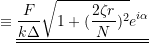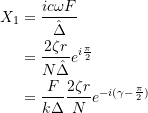### Review of single and multi-degree of freedom (mdof) systems: Complex Representation for Vibrations

When dealing with vibrating equations it is often more efficient to use complex numbers as they allow the phase relationships to be easily handled. To do this use the complex number: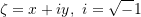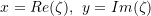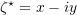is called the complex conjugate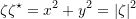Alternatively we write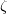as: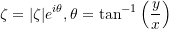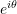is called a unit phasor. For fractional expressions we can rationalize the denominator to separate real & imaginary parts: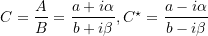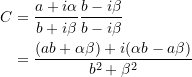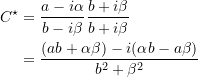Consider the steady-state forced SDOF system: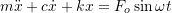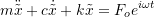and we want to imaginary part of the solution

Try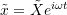, where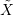is complex, therefore: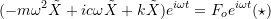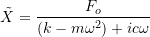Note: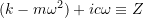,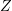is defined as the mechanical impedence.

Rationalizing the denominator gives: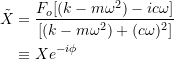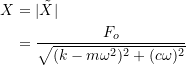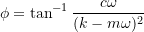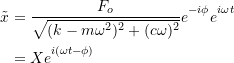as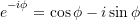Then the imaginary part of the solution is: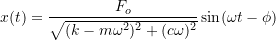We can now re-interpret equation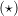as: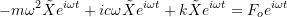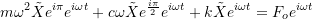Each of the force terms above can be interpreted in their respective phases.

Many real automotive suspension systems use the so-called Macpherson strut which is a combined spring and damper along with a coil spring as shown in the diagram. The system can be represented by the diagram where the forcing function comes from road surface. (This system is sometimes called a 1 &DOF system).

a) Using the mechanical impedance to show that the displacementand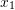.

b) For the model shown, determine the transmissibility of the system.

c) For the frequency ratio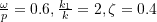, and for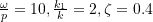. Calculate for the transmissibility and compare the values to the case in which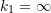.

a)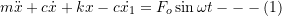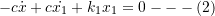Using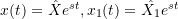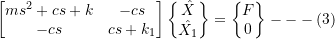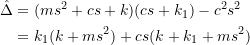But (3) is of the form: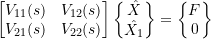Therefore: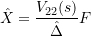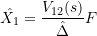If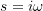: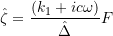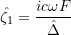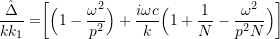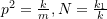, if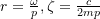: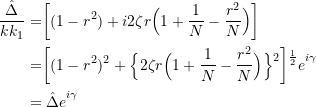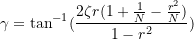If we set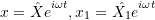,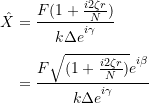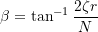Therefore,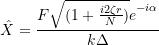Where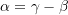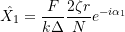Where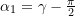b) The force transmitted is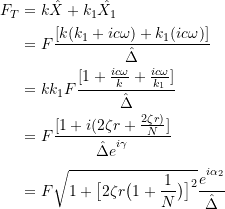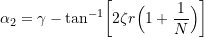c) For the case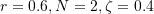: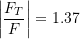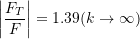For the case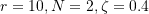: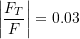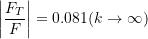The added spring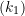reduces the transmissivity in both cases, however it is most effective at higher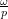ratio.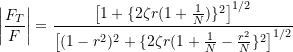If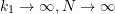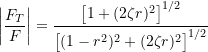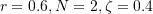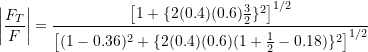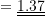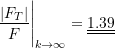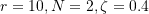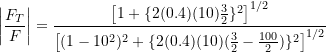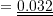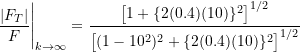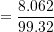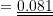## Extra Details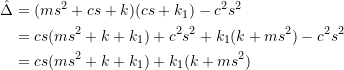If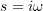: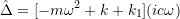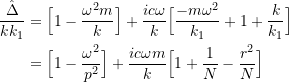Where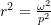,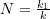, and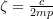. Thus: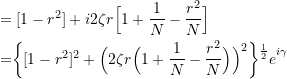Where: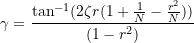If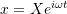,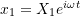: